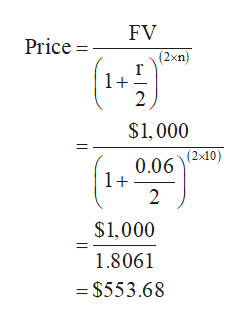# Calculate the price of a zero-coupon bond that matures in 10 years if the market interest rate is 6 percent. (Assume semiannual compounding and \$1,000 par value.)A.) \$553.68B.) \$558.66C.) \$940.00D.) \$1,000.00

Question

Calculate the price of a zero-coupon bond that matures in 10 years if the market interest rate is 6 percent. (Assume semiannual compounding and \$1,000 par value.)

A.) \$553.68

B.) \$558.66

C.) \$940.00

D.) \$1,000.00

check_circleExpert Solution
Step 1

Formula:

Step 2

Computation of zero-coupon bond price:

Hence, the price of the zero-coupon bond is \$553.68

...help_outlineImage TranscriptioncloseFV Price = (2xn) 1+ 2 \$1,000 (2x10) 0.06 1+ 2 \$1,000 1.8061 =\$553.68 fullscreen

### Want to see the full answer?

See Solution

#### Want to see this answer and more?

Solutions are written by subject experts who are available 24/7. Questions are typically answered within 1 hour*

See Solution
*Response times may vary by subject and question
Tagged in